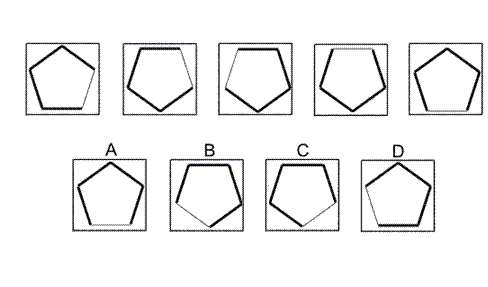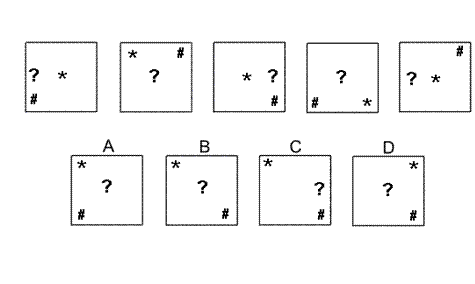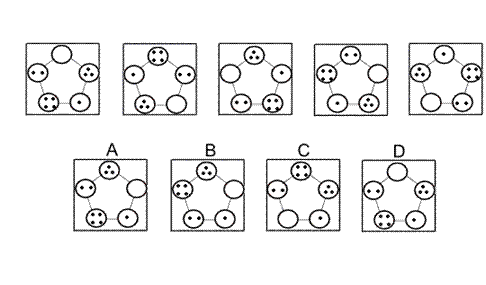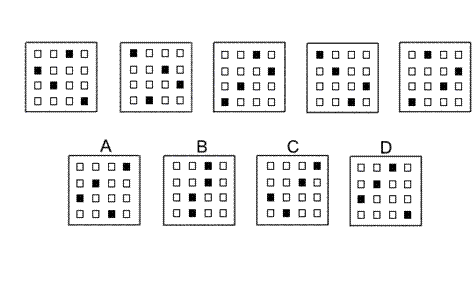Aptitude Tests 4 Me

Non Verbal Logic

Detailed solution

36.Solution: A Explanation: In this question there is a regular pentagon with one edge thinner than the others. There are three rules that are applied one after the other: The first rule is a vertical flip. The second rule is a horizontal flip. The third rule is a rotation of 72° about the centre. The second rule needs to be applied next, therefore, and the correct answer is A.

37.Solution: B Explanation: In this question the three shapes each have their own rule to follow and move around the square in an imaginary 3 by 3 grid. The question mark (?) always stays in the middle row of the 3 by 3 grid and moves one place each time, changing direction when it reaches an edge of the square. The asterisk (*) always stays on the diagonal going from the top left of the square to the bottom right, and moves one place each time, changing direction when it reaches a corner of the square. The hash symbol (#) moves clockwise around a triangle with vertices at the bottom left, top right and bottom right corners of the square. Following these rules, the next diagram in the sequence is B.

38.Solution: D Explanation: In this question there are two rules to follow. The first rule is that the pentagonal shape rotates through 72° anticlockwise each time. The second rule is that, at the same time, the number of dots increases by 1, with 4 dots becoming 0 dots. When both these rules are applied simultaneously, the next diagram in the sequence is D.

39.Solution: A Explanation: In this question there are two rules that are applied alternately. The first rule is that the first and third columns interchange and the second and fourth columns interchange. The second rule is that the rows move up one place, with the top row going to the bottom row. To find the next diagram in the sequence, the first rule must be applied next. The correct answer, therefore, is A.

 1 2 3 4 5 6 7 8 9 10 11 12 13 14 15 16 17 18 19 20 21 22 23 24 25 26 27 28 29 30 31 32 33 34 35 36 37 38 39 40 41 42 43 44 45 46 47 48 49 50 51 52 53 54 55 56 57 58 59 60 61 62 63 64 65 66 67 68 69 70 71 72 73 74 75 76 77 78 79 80 81 82 83 84 85 86 87 88Passage ReadingVerbal LogicNon Verbal LogicNumerical LogicData InterpretationReasoningAnalytical AbilityBasic NumeracyAbout UsContactPrivacy PolicyMajor TestsFAQ